JEE  >  Revisal Problems (Past 13 Years) JEE Advanced (Thermodynamics)

# Revisal Problems (Past 13 Years) JEE Advanced (Thermodynamics)

Test Description

## 20 Questions MCQ Test Chemistry for JEE Advanced | Revisal Problems (Past 13 Years) JEE Advanced (Thermodynamics)

Revisal Problems (Past 13 Years) JEE Advanced (Thermodynamics) for JEE 2023 is part of Chemistry for JEE Advanced preparation. The Revisal Problems (Past 13 Years) JEE Advanced (Thermodynamics) questions and answers have been prepared according to the JEE exam syllabus.The Revisal Problems (Past 13 Years) JEE Advanced (Thermodynamics) MCQs are made for JEE 2023 Exam. Find important definitions, questions, notes, meanings, examples, exercises, MCQs and online tests for Revisal Problems (Past 13 Years) JEE Advanced (Thermodynamics) below.
 1 Crore+ students have signed up on EduRev. Have you?
Revisal Problems (Past 13 Years) JEE Advanced (Thermodynamics) - Question 1

### Direction (Q. Nos. 1-7) This section contains 7 multiple choice questions. Each question has four choices (a), (b), (c) and (d), out of which ONLY ONE option is correct. Given,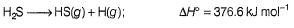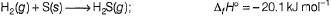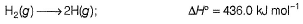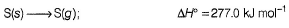Q. Thus, ΔfH° (HS) is

Revisal Problems (Past 13 Years) JEE Advanced (Thermodynamics) - Question 2

### I and II are two isotherms, between p and V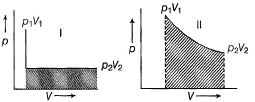Select correct alternate

Revisal Problems (Past 13 Years) JEE Advanced (Thermodynamics) - Question 3

### A 50.0 kg person drinks 500 g of milk which has a calorific value of approximately 720 cal g-1. If only 10% of the energy in milk is converted to mechanical work, how high (in meters) can the person climb based on this energy intake?

Revisal Problems (Past 13 Years) JEE Advanced (Thermodynamics) - Question 4

For the reaction,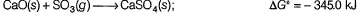Q. ΔG for this reaction at 298 K when the pressure of SO3 is 0.5 atm is

Revisal Problems (Past 13 Years) JEE Advanced (Thermodynamics) - Question 5

If the internal energy of a system decreases by 125 J and at the same time it absorbs 54 J of heat then

Revisal Problems (Past 13 Years) JEE Advanced (Thermodynamics) - Question 6

In compressing a gas, 355 J of work is done on the system. At the same time 185 J of heat escapes from the system. Thus, ΔE for the system is

Revisal Problems (Past 13 Years) JEE Advanced (Thermodynamics) - Question 7

0.4 mole of xenon is heated from 300 K to 400 K. Thus, change in internal energy is approximately (assume ideal behaviour and heat capacity is independent of temperature)

Revisal Problems (Past 13 Years) JEE Advanced (Thermodynamics) - Question 8

Direction (Q. No. 8) This section is based on Statement I and Statement II. Select the correct answer from the codes given below.

Q.

Statement I : When liquid water freezes under constant pressure, work is done by the system.

Statement II : When liquid water freezes, volume increases.

*Multiple options can be correct
Revisal Problems (Past 13 Years) JEE Advanced (Thermodynamics) - Question 9

Direction (Q. Nos. 9-11) This section contains 3 multiple choice questions. Each question has four choices (a), (b), (c) and (d), out of which ONE or MORE THAN ONE are correct.

Q. The standard enthalpies of combustion of fumaric acid and maleic acid are -1336.0 and -1359.2 kJ mol-1

ΔfH° (CO2) = - 393.5 kJ mol-1
ΔfH° (H2O) = - 285.8 kJ mol-1

*Multiple options can be correct
Revisal Problems (Past 13 Years) JEE Advanced (Thermodynamics) - Question 10

Select correct alternates based on the following experiment.

A quantity of 0.850 mole of an ideal gas initially at a pressure 15.0 atm and 300 K is allowed to expand isothermally until its final pressure is 1.00 atm. Work done

*Multiple options can be correct
Revisal Problems (Past 13 Years) JEE Advanced (Thermodynamics) - Question 11

A quantity of 200 mL of 0.862 M HCI is mixed with 200 mL of 0.431 M Ba(OH)2 in a constant pressure calorimeter that has a heat capacity of 453 JK-1. The initial temperature of the HCI and Ba(OH)2 solution is the same at 20.48°C. For the process the heat of neutralisation for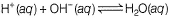is -56.2 kJ mol-1.

Select correct statement(s)

Revisal Problems (Past 13 Years) JEE Advanced (Thermodynamics) - Question 12

Direction (Q. Nos. 12-17) This section contains 3 paragraphs each describing theory, experiments, data etc. Six questions related to the paragraphs have been given. Each question has only one correct answer among the four given options (a), (b), (c) and (d).

Passage I

The combustion of methane gas, the principal constituent of natural gas is represented by the equation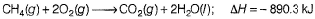Q. Quantity of heat, in kilojoules, liberated in the complete combustion of 1.65 x 104 L of CH4(g) measured at 27°C and 760 mm Hg is

Revisal Problems (Past 13 Years) JEE Advanced (Thermodynamics) - Question 13

Passage I

The combustion of methane gas, the principal constituent of natural gas is represented by the equationQ. Volume of water, in litres, that could be heated from 8.8°C to 68.8°C as a result of above heat is (density = 1 g/mL

Revisal Problems (Past 13 Years) JEE Advanced (Thermodynamics) - Question 14

Passage II

A system consisting of 1 mole of water vapour H2O(g) at 100°C and 1 atm with a volume of 30.12 L and condenses at a constant pressure of 1 atm to liquid water, H2O(l) at 100°C and 1 atm with a volume of 18.8 cm3.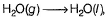ΔH = -40.7 kJ

Q. Work done in this transformation is

Revisal Problems (Past 13 Years) JEE Advanced (Thermodynamics) - Question 15

Passage II

A system consisting of 1 mole of water vapour H2O(g) at 100°C and 1 atm with a volume of 30.12 L and condenses at a constant pressure of 1 atm to liquid water, H2O(l) at 100°C and 1 atm with a volume of 18.8 cm3.ΔH = -40.7 kJ

Q. ΔE of the process is

Revisal Problems (Past 13 Years) JEE Advanced (Thermodynamics) - Question 16

Passage III

Based on the following figure.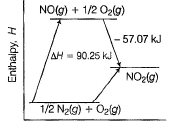Q. ΔfH of NO2(g) is

Revisal Problems (Past 13 Years) JEE Advanced (Thermodynamics) - Question 17

Passage III

Based on the following figure.Q. Heat of combustion of NO(g) is

Revisal Problems (Past 13 Years) JEE Advanced (Thermodynamics) - Question 18

Direction (Q. No. 18) Choices for the correct combination of elements from Column I and Column II are given as options (a), (b), (c) and (d), out of which one is correct.

Q. Acetic acid, CH3CO2H can form dimer (CH3CO2H)2 in the gas phase.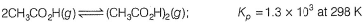The dimer is held together by two hydrogen bonds with a total strength of 66.5 kJ mol-1 of dimer.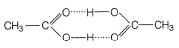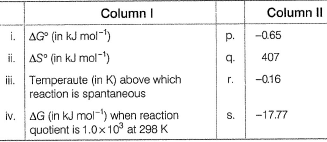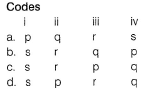*Answer can only contain numeric values
Revisal Problems (Past 13 Years) JEE Advanced (Thermodynamics) - Question 19

Direction (Q. Nos. 19 and 20) This section contains 2 questions. Each question, when worked out will result in integer from Oto 9 (both inclusive).

Q. A quantity of 0.50 mole of an ideal gas at 300 K expands isothermally against a constant pressure of 2.0 atm from 1.0 L to 5.0 L. Thus ΔS (universe) is .......

*Answer can only contain numeric values
Revisal Problems (Past 13 Years) JEE Advanced (Thermodynamics) - Question 20

The molar heat capacity of oxygen at constant pressure is given by (25.7T + 0.01307) 10-3 kJ mol-1.

Calculate the enthalpy change (in kJ) when 1.45 moles of O2 are heated from 298 K to 367 K.(Scan QR code)